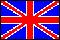ISSN 2079-3537

Scientific Visualization
 Issue Year: 2014 Quarter: 2 Volume: 6 Number: 2 Pages: 34 - 42
 Article Name: VISUALIZATION OF CALCULATION OF MINIMAL AREA SURFACES Authors: A. Klyachin (Russian Federation), V. Klyachin (Russian Federation), E. Grigorieva (Russian Federation) Address: A. Klyachin klyachin-aa@yandex.ru Volgograd State University, Volgograd, Russian Federation   V. Klyachin klchnv@mail.ru Volgograd State University, Volgograd, Russian Federation   E. Grigorieva e_grigoreva@mail.ru Volgograd State University, Volgograd, Russian Federation Abstract: This work is devoted to the numerical calculation and visualization of surfaces having the smallest area with a fixed boundary. The corresponding differential equation is nonlinear, so find an exact solution of the boundary value problem often is not possible. This leads to the problem of the development of effective methods for the approximate solution of the corresponding equation - the minimal surface equation. An important problem is to study mathematical methods found in terms of stability and convergence of approximate solutions. In this direction, we obtained the following results: - We have studied some mathematical aspects of the calculation formulas and diagrams. Made some steps to justify the methods used an approximate solution of the minimal surface equation. - On the basis of this method approximation of differential equations we obtain calculation scheme of piecewise linear surface having a minimum area of the same surfaces with a common boundary. For this calculation scheme we developed several software modules to obtain calculation results in a convenient form for subsequent processing. We done the test calculations of minimal surfaces for different spatial contours consisting of straight, parabolic and circular segments. - The developed program is based on the library OpenGL and it allows the visualization of three-dimensional data with the ability to specify how to fill individual triangles model. It was developed an experimental module MinSurf.py, which integrates into Blender. This module is implemented class system designed for storing models of minimal surfaces and implementation of such actions as: deformation of the surface model, automatically creating keyframes to animate the surface deformation, staining surfaces models based on technology UV-texturing. Language: English

 Open Article Open Articlein Russian translation Download ZIP archive Download ZIP archivein Russian translation